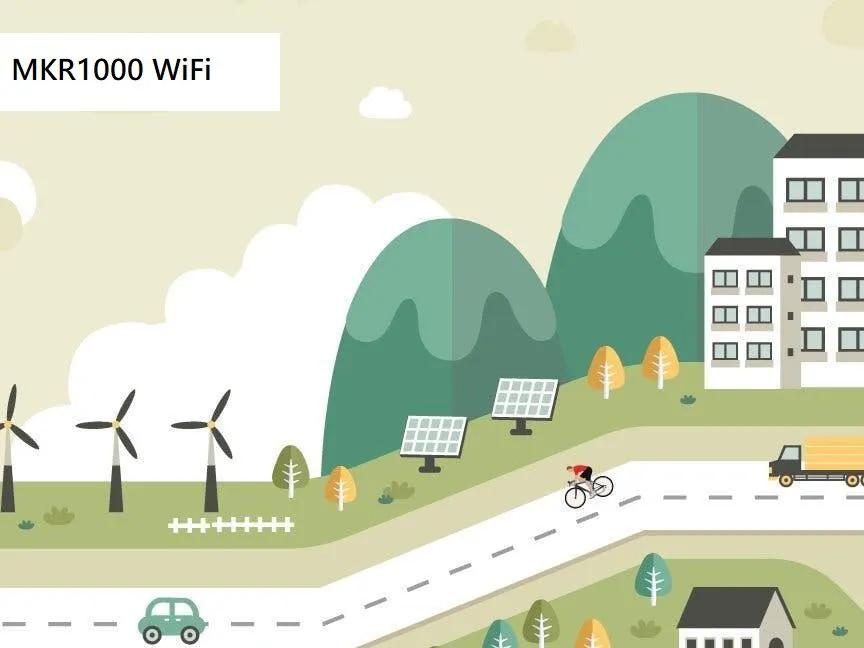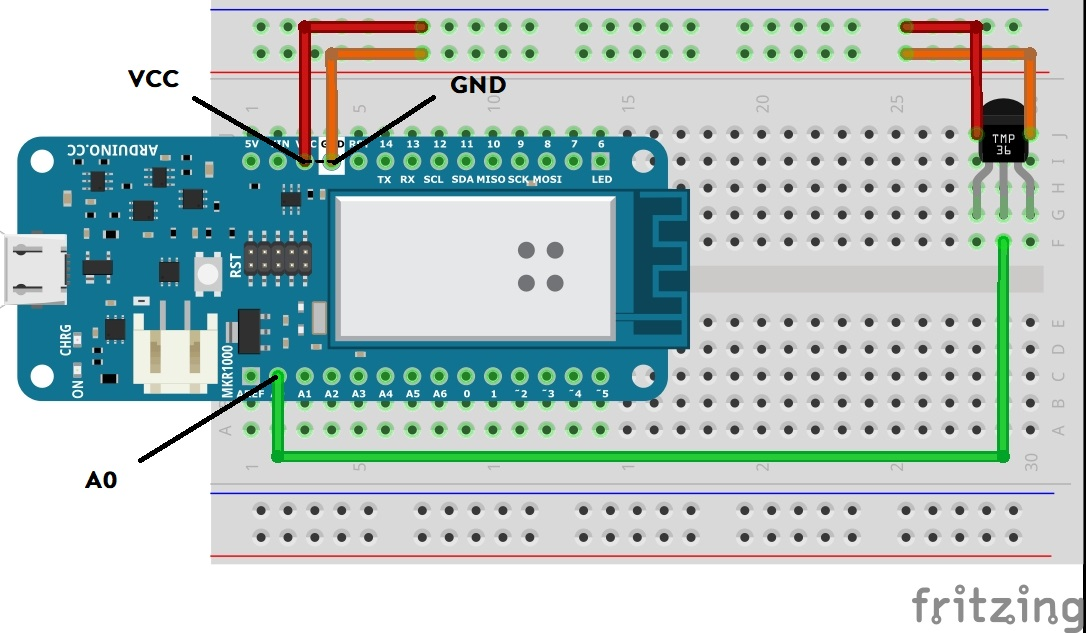Project tutorial# Connecting to the Arduino IOT Cloud (S1.2) © GPL3+

Connecting your Arduino to the Arduino IOT Cloud and display the temperature on the Cloud.

• 3,074 views
• 2 respects

## Apps and online services

### Introduction:

In this hands on lab, you will build and connect you temperature sensing device to the Arduino IOT Cloud.

This is the second in a series of labs in the SMART Cities Program and introduces a simple application of the Internet of Things with the ability to send data across the Internet to the Cloud.

### Putting it together:

There are five parts to this tutorial:

• Installing the Arduino Create plugin
• Assembling the circuit
• Setting up your board in the cloud
• Setting up the IOT Cloud

### Part 1 - Installing the Arduino Create plugin

A quick tutorial for installing the plugin can be found Here

### Part 2 - Assembling the circuit

Using the below wiring diagram as a reference assemble your device

Note:

• The temperature sensor (TMP36) is connected to pin A0
• When inserting the Temperature sensor make sure that the flat side is facing towards you.

### Part 3 - Setting up your board in the cloud

To configure your board navigate to Arduino Create and complete the following steps (highlighted in orange)

You will now see your MKR1000 board listed and named "MyThing" as shown below (circled in orange)

This completes the registration of the board in the cloud

### Part 4 - Setting up the IOT Cloud

With the device registered in the cloud navigate to Arduino Create and complete the following steps (highlighted in orange)

This completes the setup of the device in the Arduino IOT Cloud.

### Part 5 - Adding your code

Step 1 -On line 18 enter the Temperature Sensor variables (Snippet 1)

``````// Temperature Sensor variables
int sensorPin = A0;
int sensorValue = 0;
int Dres = 1024;
int VCC = 3300;          // set VCC voltage milliVolts
int offset = 500;        // set tolerance of 500 milliVolts
int scaling = 10;        // set the voltage scaling at 10mM / deg C
float voltage = 0.0;     // set the initial voltage to 0
``````

Step 2 - In void setup () on line 36 add the following setup functions (Snippet 2)

``````// Set 10bit read resolution
``````

Step 3 - In On line 59 add the main code (Snippet 3)

`````` // read the value from the sensor:
Serial.print("sensorValue = ");
Serial.print(sensorValue);
// Calculate the voltage
voltage = sensorValue * (VCC/Dres); // milliVolts
Serial.print(" voltage = ");
Serial.print(voltage);
Serial.print(" VCC = ");
Serial.print(VCC);
// Calculate the temperature
temperature = (voltage - offset ) / scaling;
Serial.print(" temperature(C) = ");
Serial.println(temperature);
delay(1000);
``````

Step 4 - Navigate to the Secret tab, enter your Wifi Network Name and password

Step 5 - Save and Verify the code

Step 6 - Upload the code

You have now completed the coding of your device !!!

### The Final Result

On completion the device will display the temperature on the Arduino IOT Cloud dashboard.

## Code

##### Snippet 1Arduino
Temperature Sensor variables
```// Temperature Sensor variables
int sensorPin = A0;       // Sets A0 as the input pin for the sensor
int sensorValue = 0;      // initialise a variable to store the value coming from the sensor
int Dres = 1024;          // set max 10-bit digital value
int VCC = 3300;           // set VCC voltage milliVolts
int offset = 500;         // set tolerance of 500 milliVolts
int scaling = 10;         // set the voltage scaling at 10mM / deg C
float voltage = 0.0;      // set the initial voltage to 0
```
##### Snippet 2Arduino
Board setup instructions
```  // Set 10bit read resolution
```
##### Snippet 3Arduino
Main program code
```  // read the value from the sensor:
Serial.print("sensorValue = ");
Serial.print(sensorValue);

// Calculate the voltage
voltage = sensorValue * (VCC/Dres); // milliVolts
Serial.print(" voltage = ");
Serial.print(voltage);
Serial.print(" VCC = ");
Serial.print(VCC);

// Calculate the temperature
temperature = (voltage - offset ) / scaling;
Serial.print(" temperature(C) = ");
Serial.println(temperature);

delay(1000);
```

## Schematics#### Author

• Author by Paynter Technology

August 30, 2020

#### Members who respect this project

See similar projects
you might like

#### An IoT controlled temperature monitor (S1.3)

Project tutorial by greg

• 4,853 views
• 3 respects

#### IoT Cloud - Getting Started

by 5 developers

• 165,618 views
• 191 respects

#### Arduino IoT Cloud Amazon Alexa Integration

Project tutorial by 4 developers

• 38,165 views
• 89 respects

#### Full Control of Your TV Using Alexa and Arduino IoT Cloud

Project tutorial by 4 developers

• 18,550 views
• 27 respects

#### IoT Pressure Sensor: MKR GSM + Arduino Cloud + Google Sheets

Project tutorial by wahltharvey

• 15,397 views